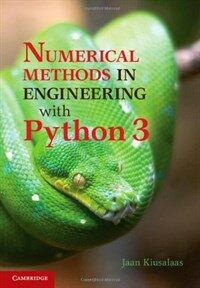> Detail View

# Detail View## Numerical methods in engineering with Python 3 (Loan 8 times)

Material type
단행본
Personal Author
Kiusalaas, Jaan.
Title Statement
Numerical methods in engineering with Python 3 / Jaan Kiusalaas.
Publication, Distribution, etc
Cambridge ;   New York :   Cambridge University Press,   c2013.
Physical Medium
xi, 423 p. : ill. ; 26 cm.
ISBN
9781107033856
요약
"This book is an introduction to numerical methods for students in engineering. It covers solution of equations, interpolation and data fitting, solution of differential equations, eigenvalue problems and optimisation. The algorithms are implemented in Python 3, a high-level programming language that rivals MATLAB in readability and ease of use. All methods include programs showing how the computer code is utilised in the solution of problems. The book is based on Numerical Methods in Engineering with Python, which used Python 2. This new edition demonstrates the use of Python 3 and includes an introduction to the Python plotting package Matplotlib. This comprehensive book is enhanced by the addition of numerous examples and problems throughout"--
General Note
Includes index.
Content Notes
Machine generated contents note: 1. Introduction to Python; 2. Systems of linear algebraic equations; 3. Interpolation and curve fitting; 4. Roots of equations; 5. Numerical differentiation; 6. Numerical integration; 7. Initial value problems; 8. Two-point boundary value problems; 9. Symmetric matrix eigenvalue problems; 10. Introduction to optimization.
Engineering mathematics --Data processing. Python (Computer program language).
 000 00000cam u2200205 a 4500 001 000045899849 005 20170317141736 008 170317s2013 enka 001 0 eng d 010 ▼a 2012036775 020 ▼a 9781107033856 035 ▼a (KERIS)REF000016987855 040 ▼a DLC ▼b eng ▼c DLC ▼e rda ▼d DLC ▼d 211009 050 0 0 ▼a TA345 ▼b .K58 2013 082 0 0 ▼a 620.00285/5133 ▼2 23 084 ▼a 620.00285 ▼2 DDCK 090 ▼a 620.00285 ▼b K62n 100 1 ▼a Kiusalaas, Jaan. 245 1 0 ▼a Numerical methods in engineering with Python 3 / ▼c Jaan Kiusalaas. 260 ▼a Cambridge ; ▼a New York : ▼b Cambridge University Press, ▼c c2013. 300 ▼a xi, 423 p. : ▼b ill. ; ▼c 26 cm. 500 ▼a Includes index. 505 8 ▼a Machine generated contents note: 1. Introduction to Python; 2. Systems of linear algebraic equations; 3. Interpolation and curve fitting; 4. Roots of equations; 5. Numerical differentiation; 6. Numerical integration; 7. Initial value problems; 8. Two-point boundary value problems; 9. Symmetric matrix eigenvalue problems; 10. Introduction to optimization. 520 ▼a "This book is an introduction to numerical methods for students in engineering. It covers solution of equations, interpolation and data fitting, solution of differential equations, eigenvalue problems and optimisation. The algorithms are implemented in Python 3, a high-level programming language that rivals MATLAB in readability and ease of use. All methods include programs showing how the computer code is utilised in the solution of problems. The book is based on Numerical Methods in Engineering with Python, which used Python 2. This new edition demonstrates the use of Python 3 and includes an introduction to the Python plotting package Matplotlib. This comprehensive book is enhanced by the addition of numerous examples and problems throughout"-- ▼c Provided by publisher. 650 0 ▼a Engineering mathematics ▼x Data processing. 650 0 ▼a Python (Computer program language). 945 ▼a KLPA

### Holdings Information

No. Location Call Number Accession No. Availability Due Date Make a Reservation Service
No. 1 Location Call Number 620.00285 K62n Accession No. 121239620 Availability In loan Due Date Make a Reservation Available for Reserve Service

### Contents information

`1. Introduction to Python; 2. Systems of linear algebraic equations; 3. Interpolation and curve fitting; 4. Roots of equations; 5. Numerical differentiation; 6. Numerical integration; 7. Initial value problems; 8. Two-point boundary value problems; 9. Symmetric matrix eigenvalue problems; 10. Introduction to optimization.`

Information Provided By: :### New Arrivals Books in Related Fields

#### Design, fabrication and characterization of multifunctional nanomaterials

Thomas, Sabu (2021)

#### 통계적 품질관리

Montgomery, Douglas C. (2022)

#### 재료과학과 공학

Callister, William D. (2021)

#### Multiscale modeling approaches for composites

Chatzigeorgiou, George (2022)

장경욱 (2022)

김도영 (2022)

#### Current research in neuroadaptive technology

Fairclough, Stephen H (2022)

이지웅 (2021)

#### 재료공학개론

Shackelford, James F. (2022)

이정원 (2022)

송철기 (2022)

이시중 (2022)

유봉조 (2022)

#### 공학수학

Zill, Dennis G. (2022)

#### (Beer의) 재료역학

Beer, Ferdinand Pierre (2022)

김태수 (2021)

김영근 (2021)

캐드앤그래픽스 (2021)

윤영삼 (2021)